# Solution assignment 04 Quadratic equations (abc-formula)

### Assignment 4

Solve: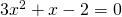### Solution

Because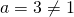we cannot easily factorize the left-hand side. So we have to apply the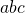-formula: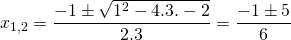The solutions are: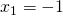or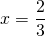0